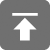# 工学1号馆

home

## Project Euler 44–Pentagon numbers

Wu Yudong    August 21, 2018     欧拉计划   561

Pentagon numbers

Pentagonal numbers are generated by the formula, Pn=n(3n−1)/2. The first ten pentagonal numbers are:

1, 5, 12, 22, 35, 51, 70, 92, 117, 145, …
It can be seen that P4 + P7 = 22 + 70 = 92 = P8. However, their difference, 70 − 22 = 48, is not pentagonal.

Find the pair of pentagonal numbers, Pj and Pk, for which their sum and difference are pentagonal and D = |Pk − Pj| is minimised; what is the value of D?

1, 5, 12, 22, 35, 51, 70, 92, 117, 145, …

// Project Euler 44–Pentagon numbers
// Completed on Tue, 21 Aug 2018, 07:35
// Language: C11
//
// 版权所有（C）wu yudong
// 博客地址：http://www.wuyudong.com

#include<stdio.h>
#include<math.h>
#include<string.h>
#include<ctype.h>
#include<stdlib.h>
#include<stdbool.h>
#include<time.h>

int pentagonal(int n)			//通过n得到五边形数
{
return n * (3 * n - 1) / 2;
}

bool judge(int n)				//判断一个数是不是五边形数
{
for (int i = 1; i * (3 * i - 1) <= 2 * n; i++) {
if (i * (3 * i - 1) == 2 * n)
return true;
}
return false;
}

void find()
{
int t1, t2;
for (int i = 2; i <= 3000; i++) {
t1 = pentagonal(i);
for (int j = 1; j < i; j++) {
t2 = pentagonal(j);
int sum = t1 + t2;
int diff = t1 - t2;
if (judge(sum) && judge(diff)) {
printf("%d\n", diff);
return;
}
}
}
}

int main()
{
int begintime, endtime;
begintime = clock();		//计时开始
find();
endtime = clock();		//计时结束
printf("Running Time: %dms\n", endtime - begintime);
return 0;
}


Running Time: 4976ms